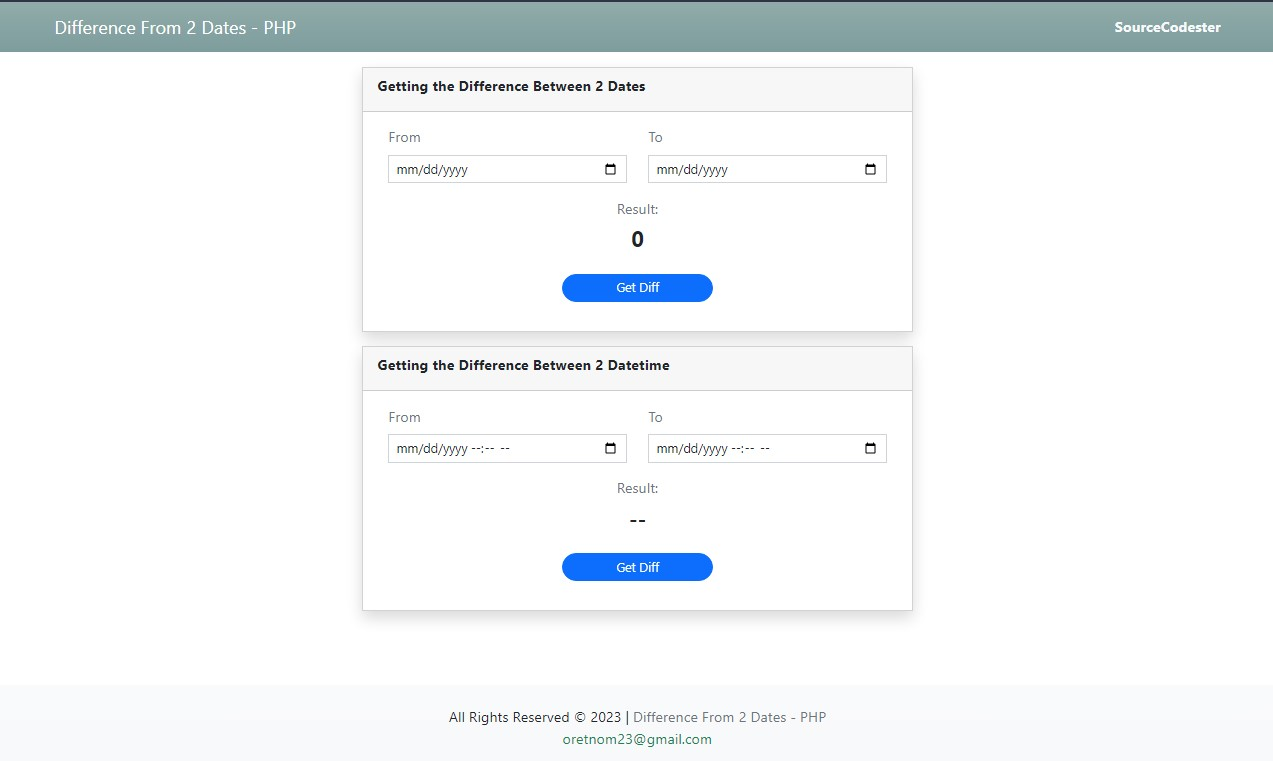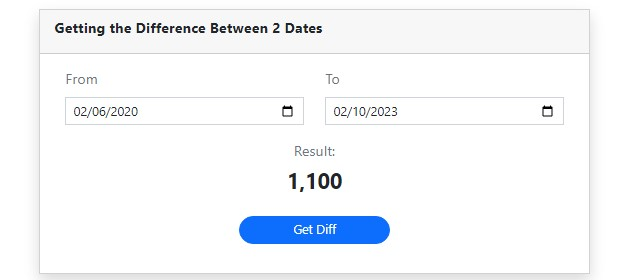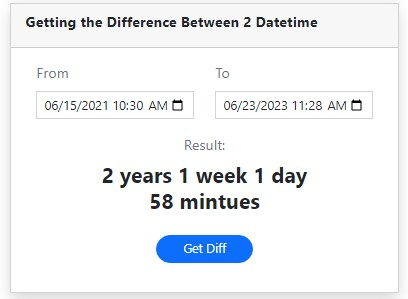# Getting the Date and Datetime Difference Between Dates in PHP Snippets

In this article, I will show you how you get the Difference between two (2) Dates or DateTime in PHP Language. The tutorial aims to provide IT/CS students and new programmers with a reference to enhance their knowledge and skills for developing an application using PHP Language. Here, snippets of some explanations are provided. A sample working source code zip file is also provided and is free to download.

## What is Date or DateTime Difference?

The Date or DateTime Difference is the gap or range between the 2 dates. In web applications, developers are usually implementing this formula or logic for getting the users' age or in any instances require the developer to get the difference between dates.

## How to Get the Difference Between 2 Dates using PHP?

Using the PHP's built-in method called strtotime(), we can simply convert the dates to timestamps which can help us to easily calculate the exact difference between the given dates. Here's an example of getting the difference if dates using the said PHP function/method:

`<?php \$date1 = "2023-06-20";\$date2 = "2023-06-23";\$diff = strtotime(\$date2) - strtotime(\$date1);\$diff = \$diff / (24 * 60 * 60);echo \$diff;//output: 3?>`

In the snippet given above, the 2 dates have been converted into timestamps using the strtotime() method and simply subtract the start date from the end date. The result will be also a timestamp and by multiplying the result by ( 24 * 60 * 60 ), the result will be converted into a day value of difference.

## How to Get the Difference Between 2 DateTime using PHP?

For getting the Difference Between 2 DateTime, we can still use the same concept that I provided in the first snippet but will use a different formula to convert the exact difference with years, months, weeks, days, hours, and minutes values. To do that, we can simply convert the timestamp differently to the said specific values using the following formula:

• Years - ( ( 365 + 365 + 365 + 365 + 366 ) / 5 )
• Months - ( ( 365 + 365 + 365 + 365 + 366 ) / 5 / 12 )
• Weeks - ( ( 365 + 365 + 365 + 365 + 366 ) / 5 / 12 / 7 )
• Days - ( ( 24 * 60 * 60 ) )
• Hours - ( ( 60 * 60 ) )
• Minutes - ( 60 )

Here's a sample snippet for getting the difference between DateTime:

`<?php     \$diff = strtotime(\$dt2) - strtotime(\$dt1);    // Getting the Year(s) Difference    \$years = floor(\$diff / (((365 + 365 + 365 + 365 + 366)/ 5) * 24 * 60 * 60));    // Getting the Motnh(s) Difference    \$months = floor((\$diff / (((365 + 365 + 365 + 365 + 366)/ 5 / 12) * 24 * 60 * 60)) - (\$years * 12));    // Getting the Week(s) Difference    \$weeks = floor((\$diff / (7 * 24 * 60 * 60)) - (\$months * (((365 + 365 + 365 + 365 + 366)/ 5) / 7) /12) - (\$years * (((365 + 365 + 365 + 365 + 366)/ 5) / 7)));     // Getting the Day(s) Difference    \$days = (\$diff / (60 * 60 * 24));    if(\$years > 0){        \$days -= (\$years * 365.2);        \$days = ceil(\$days);    }    if(\$months > 0)    \$days -= ceil(\$months * floor(365.2 / 12));    if(\$weeks > 0)    \$days -= (\$weeks * 7);    \$days = floor(\$days);     // Getting the Hour(s) Difference    \$hours = ((\$diff / ( 60 * 60)));    if(\$years > 0)    \$hours -= floor(\$years * (365 * 24));    if(\$months > 0)    \$hours -= ceil(\$months * (ceil(365.2 / 12) * 24)); // 182.6    if(\$weeks > 0)    \$hours -= ceil(\$weeks * (24* 7));    if(\$days > 0)    \$hours -= (\$days * 24);    \$hours = floor(\$hours);      // Getting the Minute(s) Difference    \$mintues = (\$diff / 60);    if(\$years > 0)    \$mintues -= (\$years * ((365 * 24) * 60));    if(\$months > 0)    \$mintues -= ceil(\$months * ((ceil(365.2 / 12) * 24))*60);    if(\$weeks > 0)    \$mintues -= (\$weeks * (24 * 7 * 60));    if(\$days > 0)    \$mintues -= (\$days * (24 * 60));    if(\$hours > 0)    \$mintues -= (\$hours * 60);     \$dt_diff_text = "";     if(\$years > 0){        if(!empty(\$dt_diff_text))  \$dt_diff_text .= " ";        \$dt_diff_text .= "{\$years} year".(\$years > 1 ? "s" : "");    }    if(\$months > 0){        if(!empty(\$dt_diff_text))  \$dt_diff_text .= " ";        \$dt_diff_text .= "{\$months} month".(\$months > 1 ? "s" : "");    }    if(\$weeks > 0){        if(!empty(\$dt_diff_text))  \$dt_diff_text .= " ";        \$dt_diff_text .= "{\$weeks} week".(\$weeks > 1 ? "s" : "");    }    if(\$days > 0){        if(!empty(\$dt_diff_text))  \$dt_diff_text .= " ";        \$dt_diff_text .= "{\$days} day".(\$days > 1 ? "s" : "");    }    if(\$hours > 0){        if(!empty(\$dt_diff_text))  \$dt_diff_text .= " ";        \$dt_diff_text .= "{\$hours} hour".(\$hours > 1 ? "s" : "");    }    if(\$mintues > 0){        if(!empty(\$dt_diff_text))  \$dt_diff_text .= " ";        \$dt_diff_text .= "{\$mintues} mintue".(\$mintues > 1 ? "s" : "");    }     echo  \$dt_diff_text;       //Output: 2 years 1 week 1 day 1 hour 29 mintues?>`

The snippets above output the exact difference between DateTime which values are converted to years, months, weeks, days, hours, and minutes values.

## Example

The below scripts will result in a simple web application that demonstrates the usage of the snippets provided above. It achieves the goal of this tutorial which is getting the difference between 2 Dates and DateTime.

### Interface

The script below is named index.php. It contains the HTML script of the application page interface.

`<?php require_once('functions.php'); if(\$_SERVER['REQUEST_METHOD'] == 'POST'){    if(isset(\$_POST['difference'])){        \$difference = get_difference(\$_POST['date1'], \$_POST['date2']);    }    if(isset(\$_POST['datetime_difference'])){        \$datetime_difference = get_datetime_difference_difference(\$_POST['dt1'], \$_POST['dt2']);    }}?><!DOCTYPE html><html lang="en"><head>    <meta charset="UTF-8">    <meta http-equiv="X-UA-Compatible" content="IE=edge">    <meta name="viewport" content="width=device-width, initial-scale=1.0">    <title>PHP - Difference From 2 Dates</title>    <link rel="stylesheet" href="https://cdnjs.cloudflare.com/ajax/libs/font-awesome/6.2.0/css/all.min.css" integrity="sha512-xh6O/CkQoPOWDdYTDqeRdPCVd1SpvCA9XXcUnZS2FmJNp1coAFzvtCN9BmamE+4aHK8yyUHUSCcJHgXloTyT2A==" crossorigin="anonymous" referrerpolicy="no-referrer" />    <link rel="stylesheet" href="https://cdn.jsdelivr.net/npm/[email protected]/dist/css/bootstrap.min.css" integrity="sha384-Zenh87qX5JnK2Jl0vWa8Ck2rdkQ2Bzep5IDxbcnCeuOxjzrPF/et3URy9Bv1WTRi" crossorigin="anonymous">    <link rel="stylesheet" href="assets/css/styles.css">    <script src="https://cdnjs.cloudflare.com/ajax/libs/font-awesome/6.2.0/js/all.min.js" integrity="sha512-naukR7I+Nk6gp7p5TMA4ycgfxaZBJ7MO5iC3Fp6ySQyKFHOGfpkSZkYVWV5R7u7cfAicxanwYQ5D1e17EfJcMA==" crossorigin="anonymous" referrerpolicy="no-referrer"></script>    <script src="https://code.jquery.com/jquery-3.6.1.js" integrity="sha256-3zlB5s2uwoUzrXK3BT7AX3FyvojsraNFxCc2vC/7pNI=" crossorigin="anonymous"></script>    <script src="https://cdn.jsdelivr.net/npm/[email protected]/dist/js/bootstrap.bundle.min.js" integrity="sha384-OERcA2EqjJCMA+/3y+gxIOqMEjwtxJY7qPCqsdltbNJuaOe923+mo//f6V8Qbsw3" crossorigin="anonymous"></script> </head><body>    <main>        <nav class="navbar navbar-expand-lg navbar-dark bg-gradient">            <div class="container">                <a class="navbar-brand" href="./">Difference From 2 Dates - PHP</a>                 <div>                    <a href="https://sourcecodester.com" class="text-light fw-bolder h6 text-decoration-none" target="_blank">SourceCodester</a>                </div>            </div>        </nav>        <div id="main-wrapper">            <div class="container px-5 my-3" >                <div class="mx-auto col-lg-6 col-md-8 col-sm-12 col-xs-12">                    <div class="card rounded-0 mb-3 shadow">                        <div class="card-header rounded-0">                            <div class="card-title"><b>Getting the Difference Between 2 Dates</b></div>                        </div>                        <div class="card-body rounded-0">                            <div class="container-fluid">                                <form action="" method="POST">                                    <input type="hidden" name="difference">                                    <div class="row">                                        <div class="col-lg-6 col-md-6 col-sm-12 col-xs-12 mb-3">                                            <label for="date1" class="form-label text-muted">From</label>                                            <input type="date" class="form-control form-control-sm rounded-0" id="date1" name="date1" required="required" value="<?= isset(\$_POST['date1']) ? \$_POST['date1'] : "" ?>">                                        </div>                                        <div class="col-lg-6 col-md-6 col-sm-12 col-xs-12 mb-3">                                            <label for="date2" class="form-label text-muted">To</label>                                            <input type="date" class="form-control form-control-sm rounded-0" id="date2" name="date2" required="required" value="<?= isset(\$_POST['date2']) ? \$_POST['date2'] : "" ?>">                                        </div>                                    </div>                                    <div class="row">                                        <div class="mb-3 col-lg-6 col-md-6 col-sm-10 col-xs-12 mx-auto text-center">                                            <label for="" class="form-label text-muted">Result:</label>                                            <h4 class="text-center fw-bolder"><?= isset(\$difference) ? number_format(\$difference) : 0 ?></h4>                                        </div>                                    </div>                                    <div class="row">                                        <div class="mb-3 col-lg-4 col-md-6 col-sm-8 col-xs-12 mx-auto text-center">                                            <button class="btn btn-primary btn-sm rounded-pill w-100">Get Diff</button>                                        </div>                                    </div>                                </form>                            </div>                        </div>                    </div>                    <div class="card rounded-0 mb-3 shadow">                        <div class="card-header rounded-0">                            <div class="card-title"><b>Getting the Difference Between 2 Datetime</b></div>                        </div>                        <div class="card-body rounded-0">                            <div class="container-fluid">                                <form action="#dt_container" id="dt_container" method="POST">                                    <input type="hidden" name="datetime_difference">                                    <div class="row">                                        <div class="col-lg-6 col-md-6 col-sm-12 col-xs-12 mb-3">                                            <label for="dt1" class="form-label text-muted">From</label>                                            <input type="datetime-local" class="form-control form-control-sm rounded-0" id="dt1" name="dt1" required="required" value="<?= isset(\$_POST['dt1']) ? \$_POST['dt1'] : "" ?>">                                        </div>                                        <div class="col-lg-6 col-md-6 col-sm-12 col-xs-12 mb-3">                                            <label for="dt2" class="form-label text-muted">To</label>                                            <input type="datetime-local" class="form-control form-control-sm rounded-0" id="dt2" name="dt2" required="required" value="<?= isset(\$_POST['dt2']) ? \$_POST['dt2'] : "" ?>">                                        </div>                                    </div>                                    <div class="row">                                        <div class="mb-3 col-lg-8 col-md-10 col-sm-12 col-xs-12 mx-auto text-center">                                            <label for="" class="form-label text-muted">Result:</label>                                            <h4 class="text-center fw-bolder"><?= isset(\$datetime_difference) ? (\$datetime_difference) : "--" ?></h4>                                        </div>                                    </div>                                    <div class="row">                                        <div class="mb-3 col-lg-4 col-md-6 col-sm-8 col-xs-12 mx-auto text-center">                                            <button class="btn btn-primary btn-sm rounded-pill w-100">Get Diff</button>                                        </div>                                    </div>                                </form>                            </div>                        </div>                    </div>                </div>            </div>        </div>        <footer class="bg-gradient bg-light shadow-top py-4 col-auto">            <div class="">                <div class="text-center">                    All Rights Reserved &copy; <span id="dt-year"></span> | <span class="text-muted">Difference From 2 Dates - PHP</span>                </div>                <div class="text-center">                    <a href="mailto:[email protected]" class="text-decoration-none text-success">[email protected]</a>                </div>            </div>        </footer>    </main></body></html>`

### PHP Functions

The script below is a PHP file named functions.php. It contains the script for getting the Difference between Dates and DateTime.

`<?php function get_difference(\$date1 = "", \$date2 = ""){     if(!empty(\$date1) && !empty(\$date2)){        \$diff = strtotime(\$date2) - strtotime(\$date1);        \$diff = \$diff / (24 * 60 * 60);        return \$diff;    }    return 0;} function get_datetime_difference_difference(\$dt1 = "", \$dt2 = ""){     if(!empty(\$dt1) && !empty(\$dt2)){         \$diff = strtotime(\$dt2) - strtotime(\$dt1);        // Getting the Year(s) Difference        \$years = floor(\$diff / (((365 + 365 + 365 + 365 + 366)/ 5) * 24 * 60 * 60));        // Getting the Motnh(s) Difference        \$months = floor((\$diff / (((365 + 365 + 365 + 365 + 366)/ 5 / 12) * 24 * 60 * 60)) - (\$years * 12));        // Getting the Week(s) Difference        \$weeks = floor((\$diff / (7 * 24 * 60 * 60)) - (\$months * (((365 + 365 + 365 + 365 + 366)/ 5) / 7) /12) - (\$years * (((365 + 365 + 365 + 365 + 366)/ 5) / 7)));         // Getting the Day(s) Difference        \$days = (\$diff / (60 * 60 * 24));        if(\$years > 0){            \$days -= (\$years * 365.2);            \$days = ceil(\$days);        }        if(\$months > 0)        \$days -= ceil(\$months * floor(365.2 / 12));        if(\$weeks > 0)        \$days -= (\$weeks * 7);        \$days = floor(\$days);         // Getting the Hour(s) Difference        \$hours = ((\$diff / ( 60 * 60)));        if(\$years > 0)        \$hours -= floor(\$years * (365 * 24));        if(\$months > 0)        \$hours -= ceil(\$months * (ceil(365.2 / 12) * 24)); // 182.6        if(\$weeks > 0)        \$hours -= ceil(\$weeks * (24* 7));        if(\$days > 0)        \$hours -= (\$days * 24);        \$hours = floor(\$hours);          // Getting the Minute(s) Difference        \$mintues = (\$diff / 60);        if(\$years > 0)        \$mintues -= (\$years * ((365 * 24) * 60));        if(\$months > 0)        \$mintues -= ceil(\$months * ((ceil(365.2 / 12) * 24))*60);        if(\$weeks > 0)        \$mintues -= (\$weeks * (24 * 7 * 60));        if(\$days > 0)        \$mintues -= (\$days * (24 * 60));        if(\$hours > 0)        \$mintues -= (\$hours * 60);         \$dt_diff_text = "";         if(\$years > 0){            if(!empty(\$dt_diff_text))  \$dt_diff_text .= " ";            \$dt_diff_text .= "{\$years} year".(\$years > 1 ? "s" : "");        }        if(\$months > 0){            if(!empty(\$dt_diff_text))  \$dt_diff_text .= " ";            \$dt_diff_text .= "{\$months} month".(\$months > 1 ? "s" : "");        }        if(\$weeks > 0){            if(!empty(\$dt_diff_text))  \$dt_diff_text .= " ";            \$dt_diff_text .= "{\$weeks} week".(\$weeks > 1 ? "s" : "");        }        if(\$days > 0){            if(!empty(\$dt_diff_text))  \$dt_diff_text .= " ";            \$dt_diff_text .= "{\$days} day".(\$days > 1 ? "s" : "");        }        if(\$hours > 0){            if(!empty(\$dt_diff_text))  \$dt_diff_text .= " ";            \$dt_diff_text .= "{\$hours} hour".(\$hours > 1 ? "s" : "");        }        if(\$mintues > 0){            if(!empty(\$dt_diff_text))  \$dt_diff_text .= " ";            \$dt_diff_text .= "{\$mintues} mintue".(\$mintues > 1 ? "s" : "");        }         return \$dt_diff_text;    }    return 0;}?>`

### Snapshots

Here are the snapshots of the application interface:

#### Page Interface#### Getting the Difference Between Dates Panel/Card#### Getting the Difference Between DateTime Panel/Card